# Waves: the Essentials

3/29/07

### Preliminaries:

Be familiar with the jargon: longitudinal, transverse, traveling waves, standing waves, nodes and antinodes.  Know that experimentally a traveling wave on a string or spring keeps its shape to a good approximation.

Be able to derive the dependence of wave velocity on tension T, or bulk modulus B, density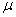or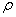using dimensional arguments.

### Waves on a String:

Thoroughly understand the figure:.

Be able to draw it and use it to derive the wave equation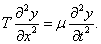The Principle of Superposition holds for solutions to the wave equation.

### Harmonic Waves:

Be familiar with the standard notation for harmonic waves:Be able to derive the expressions for both kinetic energy and potential energy per unit length in a harmonic wave on a string, and add them to find the power, the rate at which energy is moving down the string.

### Sound Waves:

Understand why the pressure in a sound wave is greatest when the displacement is least, and vice versa.

Know by heart the wave equation. (and know what B is.)

### Boundary Conditions for Pipes:

Be able to use the Principle of Superposition to figure out what happens to a single pulse wave when it reaches the end of a string or pipe – closed or open.

Know: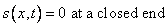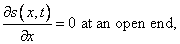and know how to use them to determine allowed frequencies of vibration of air in pipes.  Why does the lowest note in a pipe closed at one end and open at the other has a wavelength four times the length of the pipe?

### Traveling Waves: Power and Intensity:.

Power put out by a speaker emitting harmonic wave:

Power = P = rate of working = force x velocity = pressure x area x velocity

Be able to use this to derive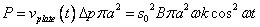, and from thatDefinition and units of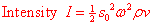.  (Watts /m2.)

### 2-D Waves

Understand the two-slit diffraction pattern, and how it can be used to find the wavelength of light.  (See the Young’s Experiment Flashlet.)   Know qualitatively how having more slits affects the pattern.

Memorize a few points on the dB scale, and their meaning in terms of power: hearing threshold, normal conversation and pain threshold.

How does the intensity of sound vary on moving away from the source?

Know the sequences of allowed harmonics in pipes with open or closed ends.

Be able to explain why two notes of almost equal frequency produce beats.

Know the formulas for the Doppler shift for sound and light, and be able to use them.